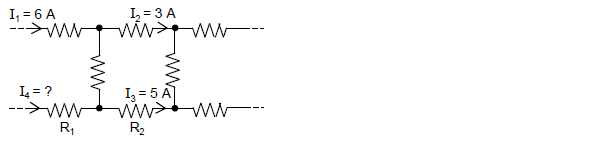Problem: A partial circuit is shown below. What is the magnitude of I4? A. 2 A B. 3 A C. 4 A D. 5 A E. 6 A

97% (69 ratings)
Problem Details

A partial circuit is shown below. What is the magnitude of I4?

A. 2 A

B. 3 A

C. 4 A

D. 5 A

E. 6 A